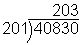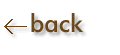Significant Figures in Derived Results:

Multiplication & Division

1)  Determine how many significant figures there are in each number to be multiplied or divided.

2)  Determine which of these is the least number of significant figures

3)  This is the number of significant digits to keep in the final answer.

The product or quotient should be rounded off to the same number of significant figures as in the measurement with the fewest significant figures.

Examples:  (The significant figures are blue.  The uncertain digit is bold.)

Multiplication                                                                      Division

 3020.1     (5 significant figures)    x      2.0     (2 sig fig)      6040.2  =   6000(3 sig fig)   (4 sig fig) "2" is the least number of significant figures.  So, keep 2 sig fig in the final answer.  The line indicates that the first zero is significant, and the last two are place holders. "3" is the least number of significant figures.  So, keep 3 sig fig in the final answer.